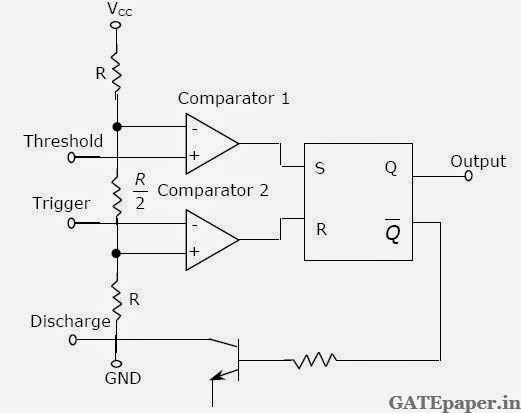### GATE 1998 ECE Analog Circuits (Analog Electronics) - Video Solutions

1. The fτ of a BJT is related to its gm, Cπ and Cµ as follows2. The circuit of the figure is an example of feedback of the following type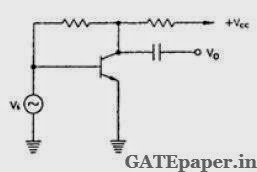a. Current Series
b. Current Shunt
c. Voltage Series
d. Voltage Shunt

3. In a differential amplifier, CMRR can be improved by using an increased
a. Emitter resistance
b. Collector resistance
c. Power supply voltages
d. Source resistance

4. From a measurement of the rise time of the output pulse of an amplifier, whose input is a small amplitude square wave, one can estimate the following parameter of the amplifier
a. Gain-bandwidth product
b. Slew rate
c. Upper 3 dB frequency
d. Lower 3 dB frequency

5. A distorted sinusoid has the amplitudes A1, A2, A3 … of the fundamental, second harmonic, third harmonic, …..respectively. The total harmonic distortion is6. The emitter coupled pair of BJT’s given a linear transfer relation between the differential output voltage and the differential input voltage Vid, only when the magnitude of Vid is less α times the thermal voltage, where α is
a. 4
b. 3
c. 2
d. 1

7. In a Shunt – Shunt negative feedback amplifier, as compared to the basic amplifier
a. Both, input and output impedances decreases
b. Input impedance decreases and output impedance increases
c. Input impedance increases and output impedance decreases
d. Both, input and output impedances increases

8. A multistage amplifier has a low pass response with three real poles at s = - ω1, - ω2 and -ω3. The approximate overall bandwidth B of the amplifier will be given by9. A high – Q quartz crystal exhibits series resonance at the frequency ωs and parallel resonance at the frequency ωp. Then
a. ωs is very close to, but less than ωp
b. ωs << ωp
c. ωs is very close to, but greater than ωp
d. ωs >> ωp

10. One input terminal of high gain comparator circuit is connected to ground and a sinusoidal voltage is applied to the other input. The output of comparator will be
a. A sinusoid
b. A full rectified sinusoid
c. A half rectified sinusoid
d. A square wave

11. In a series regulated power supply circuit, the voltage gain Av of the pass transistor satisfies the condition
a. Av -> ∞
b. 1 << Av < ∞
c. Av ≡ 1
d. Av << 1

12. For full wave rectification, a four diode bridge rectifier is claimed to have the following advantages over a two diode circuit:
1. Less expensive transformer
2. Smaller size transformer, and
3. Suitability for higher voltage application.
Of these,
a. Only 1 and 2 are true
b. Only 1 and 3 are true
c. Only 2 and 3 true
d. 1, 2 as well as 3 are true

13. In the MOSFET amplifier of the figure, the signal output V1 and V2 obey the relationship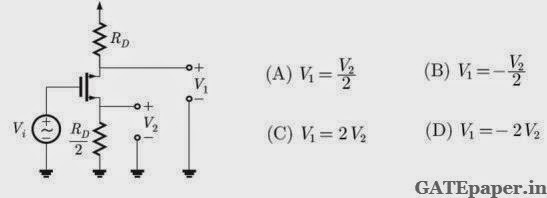14. For small signal ac operation, a practical forward biased diode can be modeled as
a. Resistance and capacitance in series
b. Ideal diode and resistance in parallel
c. Resistance and ideal diode in series
d. Resistance

15. For the circuit shown,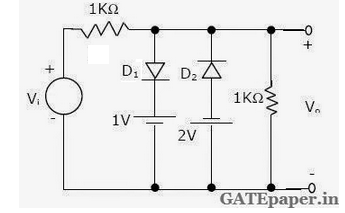a. Draw the transfer characteristics if both diodes D1 and D2 are ideal.
b. How would the characteristics change, if D2 is ideal but D1 is non-ideal? Assume D1 has forward resistance of 10 Ω and a reverse resistance of infinity.

16. Determine the input impedance of the circuit shown and investigate if it can be inductive.17. Find the value of R’ in the circuit shown for generating sinusoidal oscillations. Find the frequency of oscillations.18. In the circuit shown, determine the resistance Ro seen by the output terminals. Ignore the effect of R1 and R2.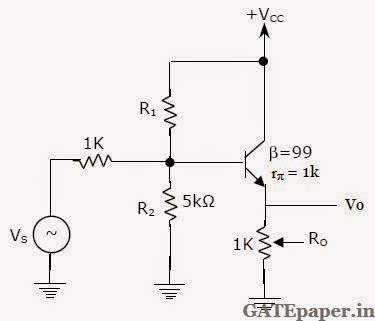19. Implement a monostable multivibrator using the timer circuit shown in figure. Also determine an expression for ON time ‘T’ of the output pulse.